## Why hash tables should use a prime-number size

I read in several books and online pages that hash tables should use a prime number for the size. Nobody really justified this statement properly. Here’s my attempt!

I believe that it just has to do with the fact that computers work with in base 2. Just think at how the same thing works for base 10:

• 8 % 10 = 8
• 18 % 10 = 8
• 87865378 % 10 = 8
• 2387762348 % 10 = 8

It doesn’t matter what the number is: as long as it ends with 8, its modulo 10 will be 8. You could pick a huge power of 10 as modulo operator, such as 10^k (with k > 10, let’s say), but

1. you would need a huge table to store the values
2. the hash function is still pretty stupid: it just trims the number retaining only the first k digits starting from the right.

However, if you pick a different number as modulo operator, such as 12, then things are different:

• 8 % 12 = 8
• 18 % 12 = 6
• 87865378 % 12 = 10
• 2387762348 % 12 = 8

We still have a collision, but the pattern becomes more complicated, and the collision is just due to the fact that 12 is still a small number.

Picking a big enough, non-power-of-two number will make sure the hash function really is a function of all the input bits, rather than a subset of them.

## Metaphysics on geometric distribution in probability theory

I realized geometric distribution is not exactly about the time needed to get the first success in a given number of trials. This is a very odd feeling. It is probably a feeling applied mathematicians get sometimes, when they feel they are doing the best they can, and yet the theory is not perfect.

This may be a naive post, I warn you, but I was really stunned when I realized this.

### Geometric distribution is not about the first success

Let’s jump to the point. We know (or at least, I was taught) that geometric distribution is used to calculate the probability that the first success in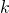trials (all independent and of probability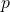) will happen precisely at the-th trial.

Remember that a geometric distribution is a random variable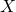such that its distribution is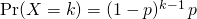How can we relate the above distribution with the fact that it matches the first success? Well, we need to have one success, which explains theat the bottom. Moreover, we want to have just one success, so all other trials must be unsuccessful, which explains the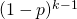.

But hey, where would first ever be written? Continue reading “Metaphysics on geometric distribution in probability theory”

## Random variables: what are they and why are they needed?

This article aims at providing some intuition for what random variables are and why random variables are useful and needed in probability theory.

## Intuition for random variables

Informally speaking, random variables encode questions about the world in a numerical way.

How many heads can I get if I flip a coin 3 times?

How many people will vote the Democrats at the US presidential elections?

I want to make pizza. What is the possible overall cost of the ingredients, considering all combinations of different brands of them?

These are all examples of random variables. What a random variable does, in plain words, is to take a set of possible world configurations and group them to a number. What I mean when I say world configurations will be clearer soon, when talking about the sample space(which, appropriately, is also called universe).

## Relationship between reduced rings, radical ideals and nilpotent elements

This post aims at providing some intuition and meaning for the following algebra relationship:

Reduced ring – Radical ideal – Nilpotent

## Reduced ring – Radical ideal – Nilpotent

A basic fact of ring theory is that if you take a ringand quotient it for a (double-sided) radical ideal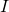you get a reduced ring. Let us suppose A is a commutative ring and understand why this fact is true.

Nilpotent element
Def.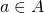is nilpotent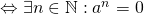Informally, a nilpotent element is like a road ending in the middle of nowhere, collapsing in the depth of an abyss. You are driving on it, following the powers of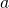, and then all of a sudden, with no explanation, your road ends in a big black hole. Indeed, the zero really acts as some kind of black hole, attracting nilpotent-made roads at some point or another: we can think of nilpotent roads as spiraling into the zero.

## Overdetermined and underdetermined systems of equations put simply

This article aims at providing real world examples and links for overdetermined and undetermined systems of equations. Before starting, we will suppose that all over and underdetermined systems are obtained from square systems which admit one and only one solution (i.e. comes from a coefficient matrix with non zero determinant).

## Overdetermined systems

When a system of linear equations has more equations than unknowns, we say it is overdetermined. It means what it says: too many rules at once are being imposed, and some of them may be conflicting. Still, it is false to say that an overdetermined system does not have any solutions: it may or it may not.

### Intuition

Intuitively, we can think of a system of equations as a set of requests. Imagine you have a group of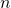people in front of you (the unknowns), and you are supposed to give each person something specific to do. If you give more commands than the number of people, then we have an overdetermined system. It is clear that when this happens, at least one person must have received more than one command. However, there are two possible scenarios.

## Quick method to find line of shortest distance for skew lines

In linear algebra it is sometimes needed to find the equation of the line of shortest distance for two skew lines. What follows is a very quick method of finding that line.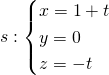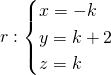So they clearly aren’t parallel. They aren’t incidental as well, because the only possible intersection point is for, but when,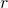is at, which doesn’t belong to. It does indeed make sense to look for the line of shortest distance between the two, confident that we will find a non-zero result.